﻿ 快堆悬浮式非能动停堆组件移动体落棒性能分析
«上一篇文章快速检索 高级检索

 应用科技2019, Vol. 46Issue (2): 116-121  DOI: 10.11991/yykj.2018040140

引用本文PENG Guannan, KUANG Bo, WANG Xin, et al. Performance analysis of a moving body in passive shutdown subassembly for sodium cooled fast reactor[J]. Applied Science and Technology, 2019, 46(2), 116-121. DOI: 10.11991/yykj.201804014.文章历史

Performance analysis of a moving body in passive shutdown subassembly for sodium cooled fast reactor
PENG Guannan, KUANG Bo, WANG Xin, LIU Pengfei, YUAN Haoran
School of Mechanical Engineering, Shanghai Jiao Tong University, Shanghai 200240, China
Abstract: In order to study the influence of the structure of passive shutdown subassembly on the moving body in a sodium cooled fast reactor (SFR), then apply the study result to guide the structural design of passive shutdown subassembly, we first analyzed the hydrodynamics and kinematics of the moving body in the hydraulic-suspended passive shutdown assembly of sodium cooled fast reactor, then designed and developed a hydrodynamic analysis code, namely PSSD, for calculating the falling performance of the moving body of passive shutdown subassembly. With the code, we made sensitivity calculation and analysis on the influence of various key dimensional sizes of the assembly, including those of the moving body on the rod-falling performance, expecting to provide a reference for the design and optimization of the passive shutdown assembly structure.
Keywords: sodium cooled fast reactor    passive shutdown subassembly    sensitivity analysis    passive safety    quasi-steady state analysis    numerical simulation    sports characteristics    control rod

1 PSS原理及移动体受力分析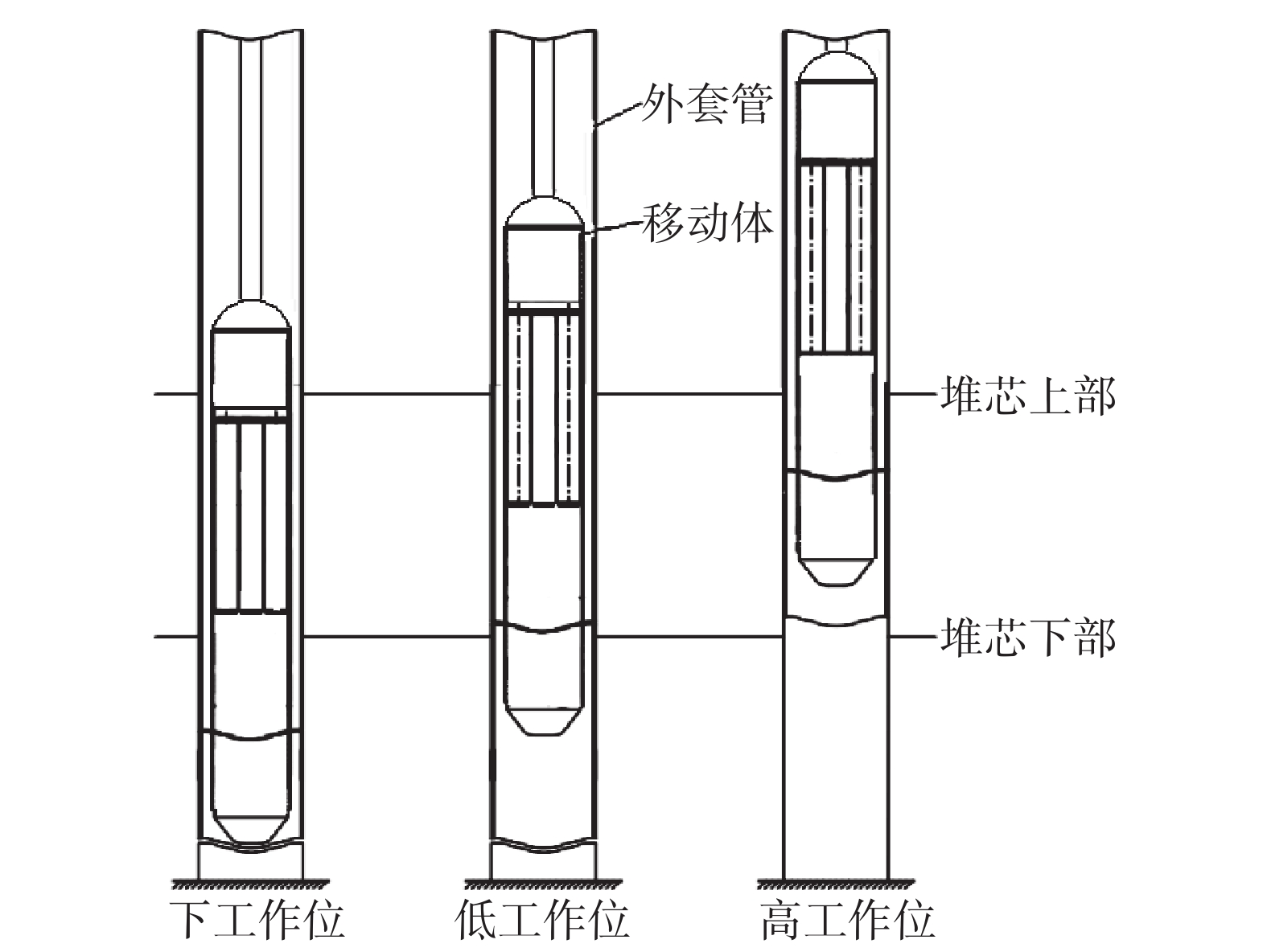Download: 图 1 PSS原理

2 移动体运动模型及计算

 $\left\{ \begin{array}{l} ma = {F_g} - {F_b} - {F_{{\rm{DR}}}}\\ x = {u_0}t + \displaystyle\frac{1}{2}a{t^2} \end{array} \right.$ (1)

 ${F_{{\rm{DR}}}} = {S_e}\Delta P$

 $\left\{ {\begin{array}{*{20}{c}} {{Q_1} - {Q_2} - {Q_5} = 0}\\ {\begin{array}{*{20}{c}} {\begin{array}{*{20}{c}} {{Q_2} + {Q_6} - {Q_3} = 0}\\ {\begin{array}{*{20}{c}} {{Q_5} - {Q_6} - {Q_7} = 0}\\ {\begin{array}{*{20}{c}} {{Q_7} + {Q_3} - {Q_4} = 0}\\ {\Delta {p_{{\rm{6}} - {\rm{7}} - {\rm{14}}}} = \Delta {p_{{\rm{6}} - 12 - 14}}} \end{array}} \end{array}} \end{array}}\\ {\Delta {p_{7 - 14 - 10}} = \Delta {p_{7 - 10}}} \end{array}} \end{array}} \right.$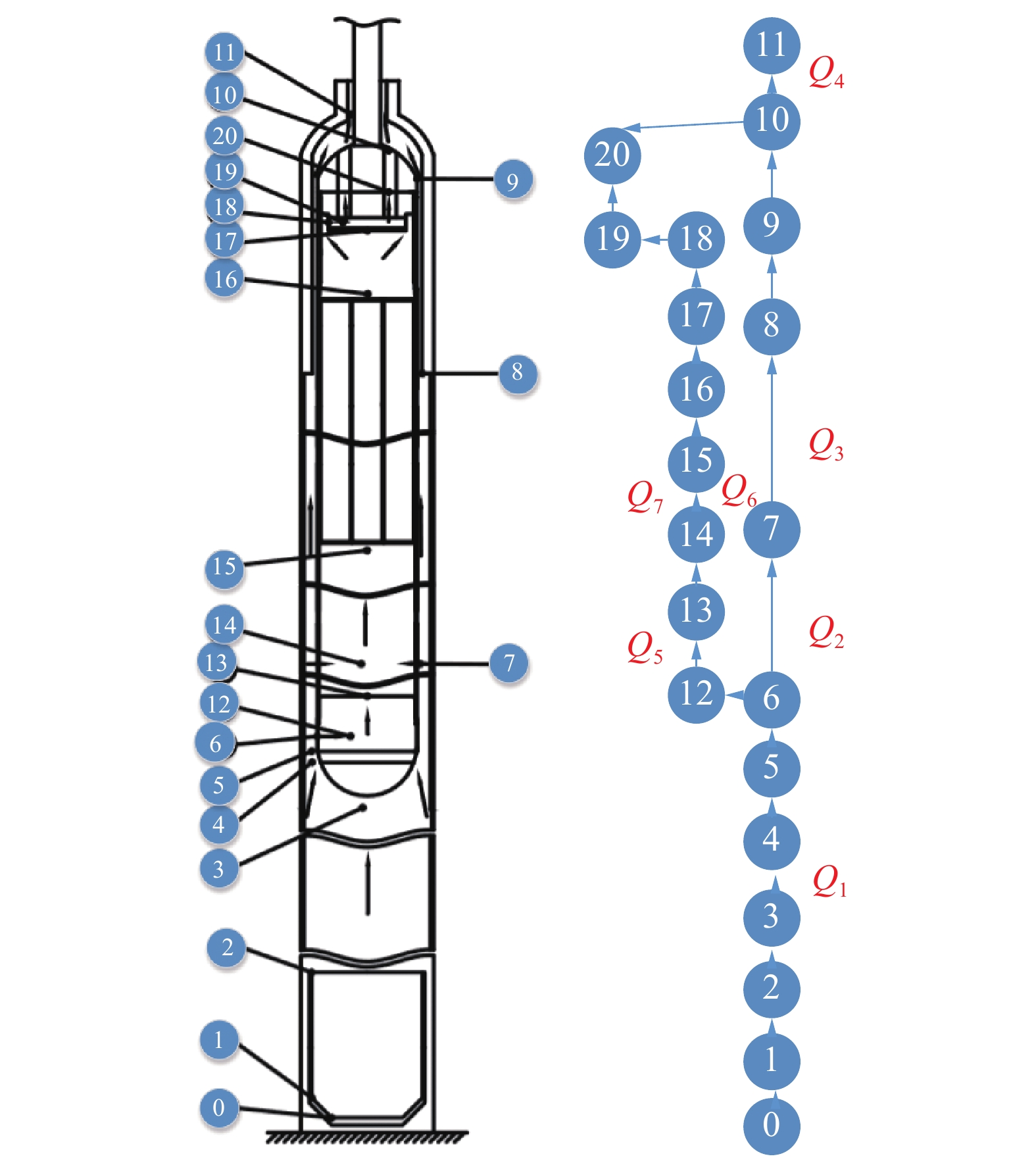Download: 图 2 流道网络

 ${\delta _p} = {f_n}\left( {\frac{1}{2}\rho u{A_n}} \right)$

 ${Q'_n} = {Q_n} + {\delta _q} = {Q_n} + \rho u{A_n}$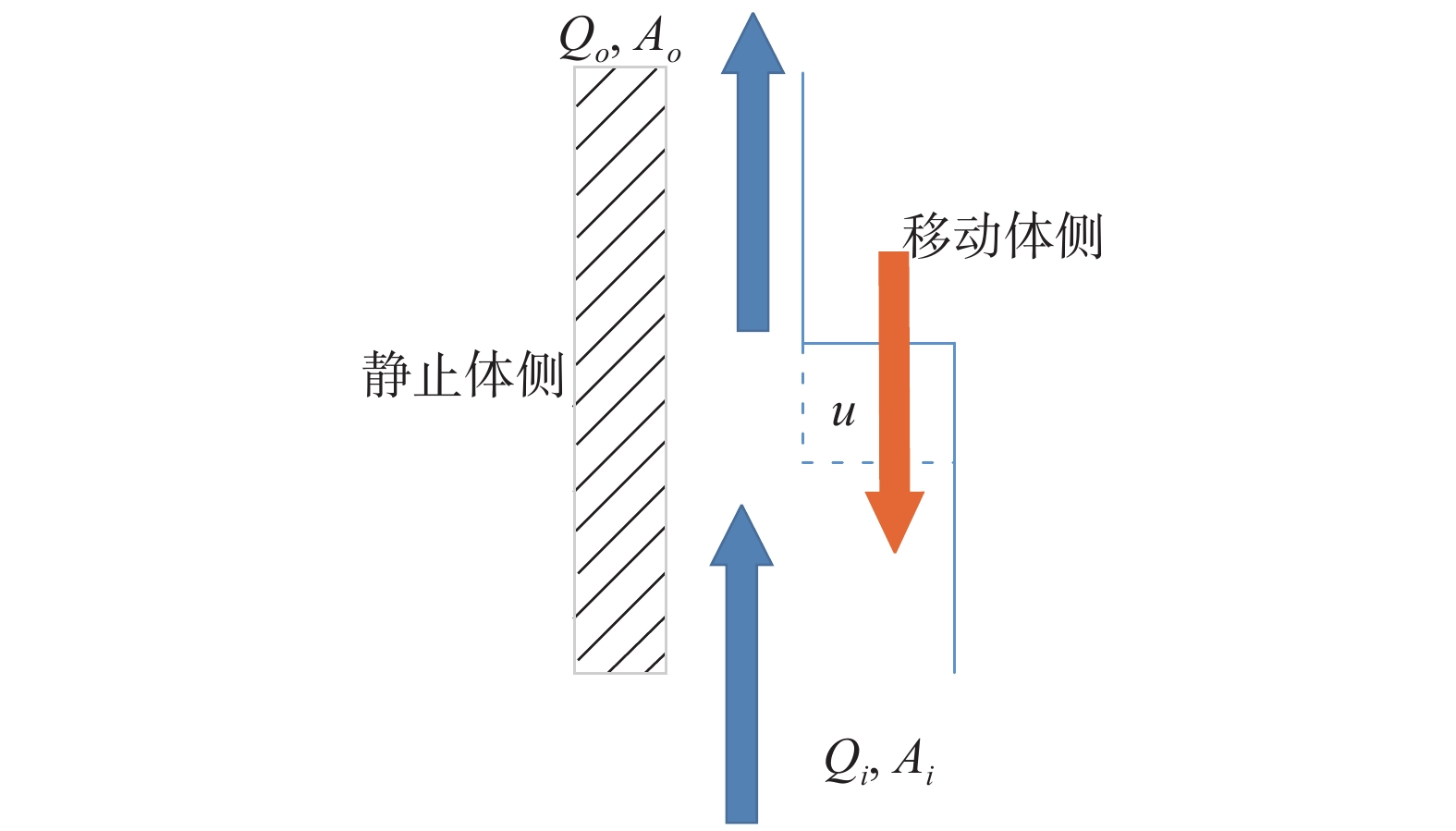Download: 图 3 移动体与外套管间突缩
3 计算流程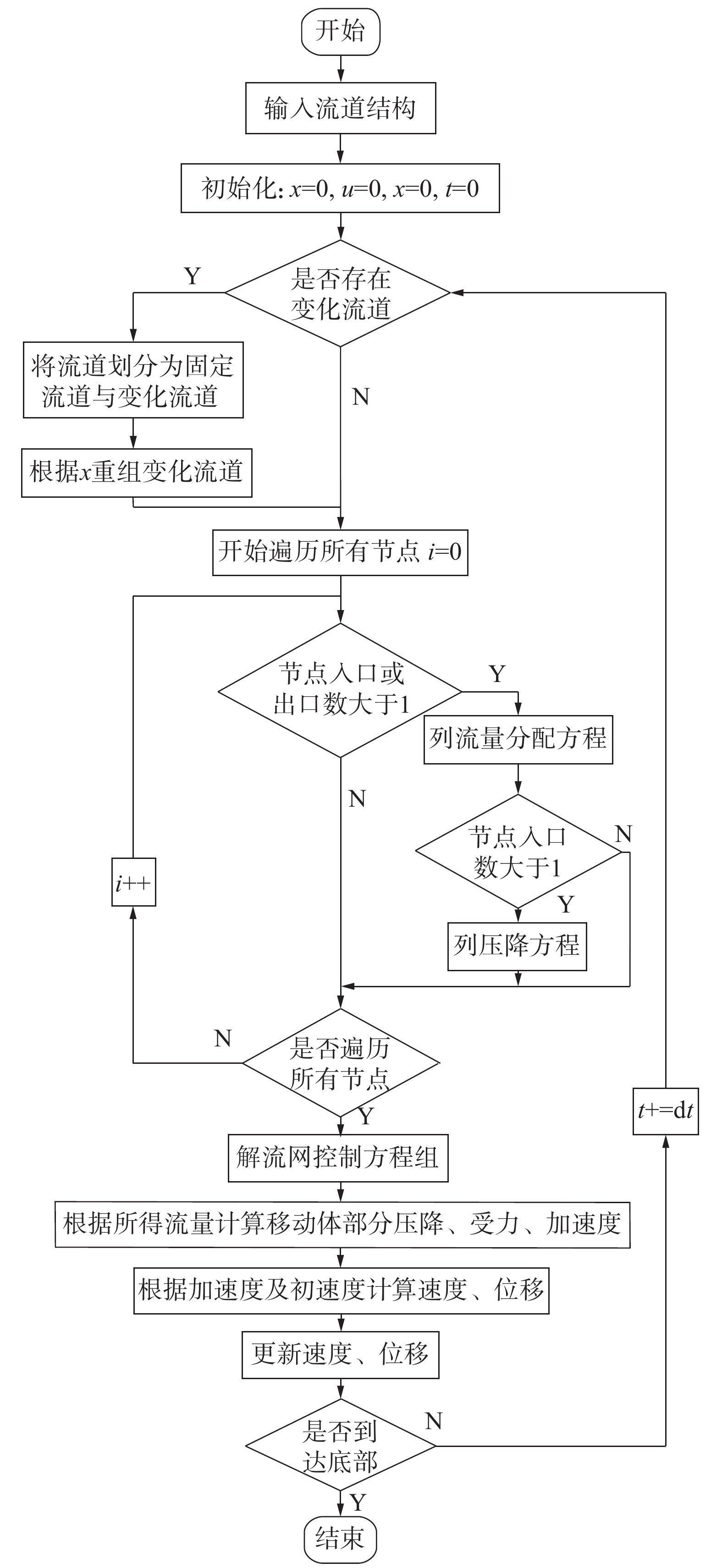Download: 图 4 计算流程
4 移动体落棒性能计算

4.1 时间步长的选择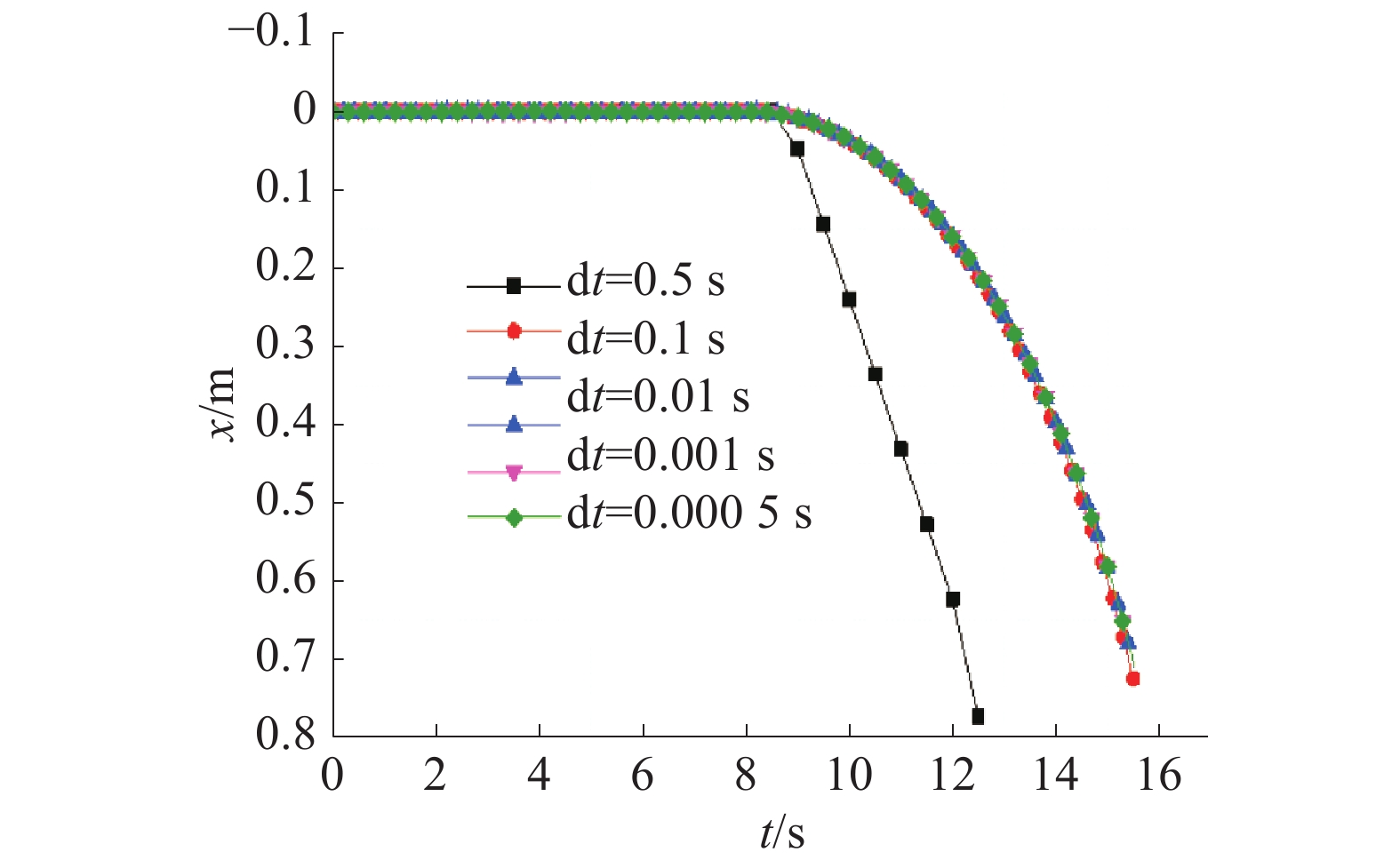Download: 图 5 不同时间步长下计算结果
4.2 基准结构尺寸移动体的落棒运动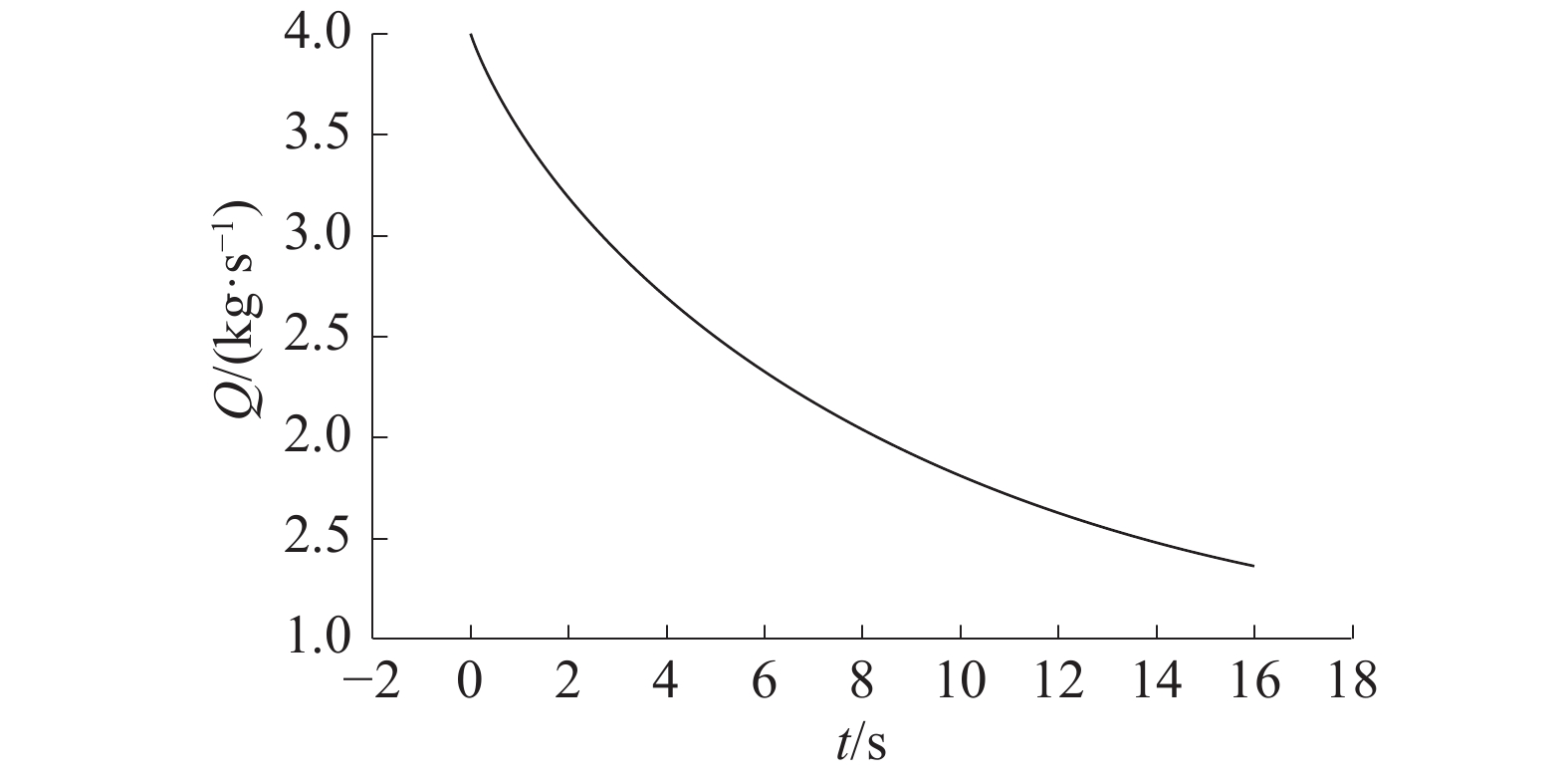Download: 图 6 入口流量曲线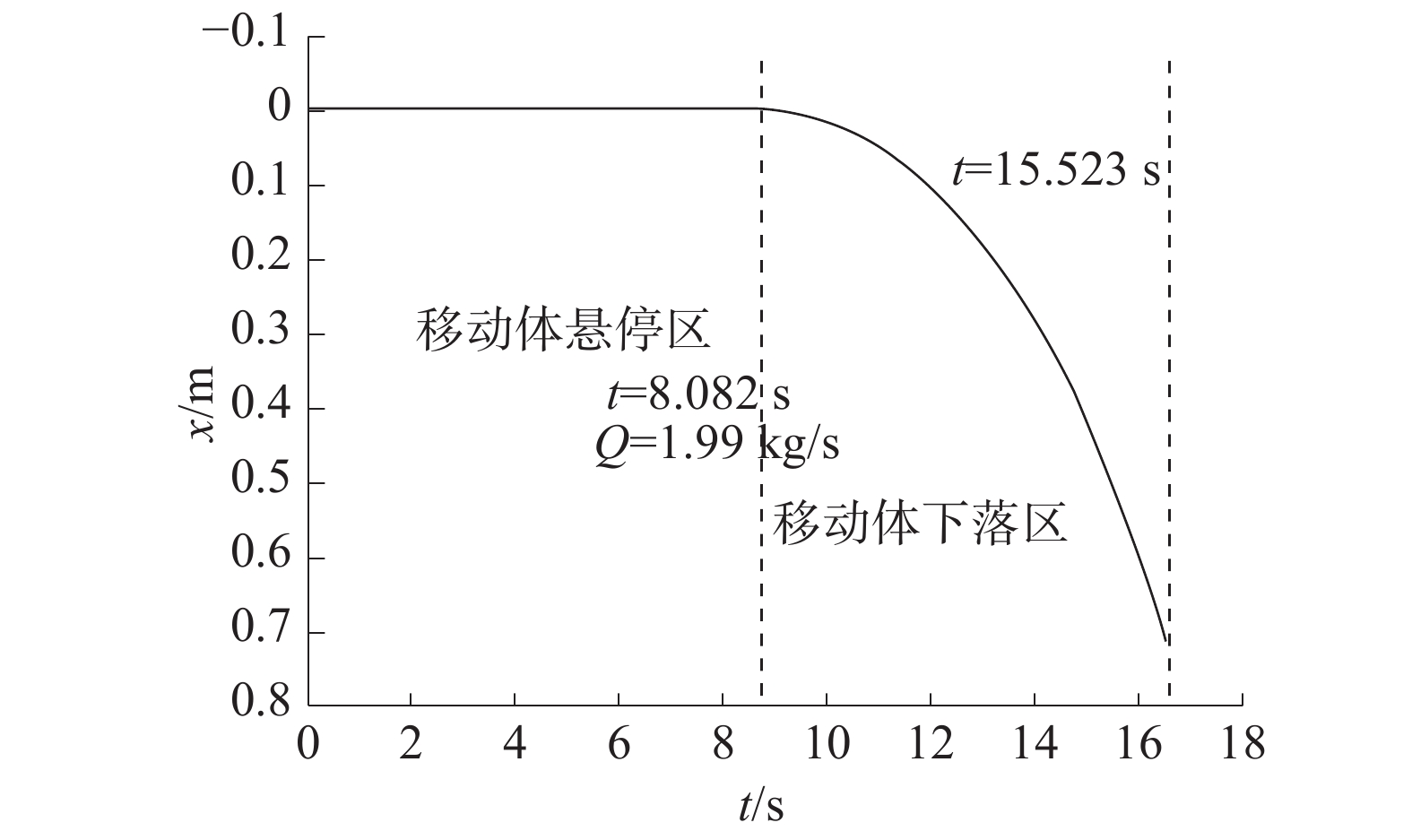Download: 图 7 标准结构下落棒曲线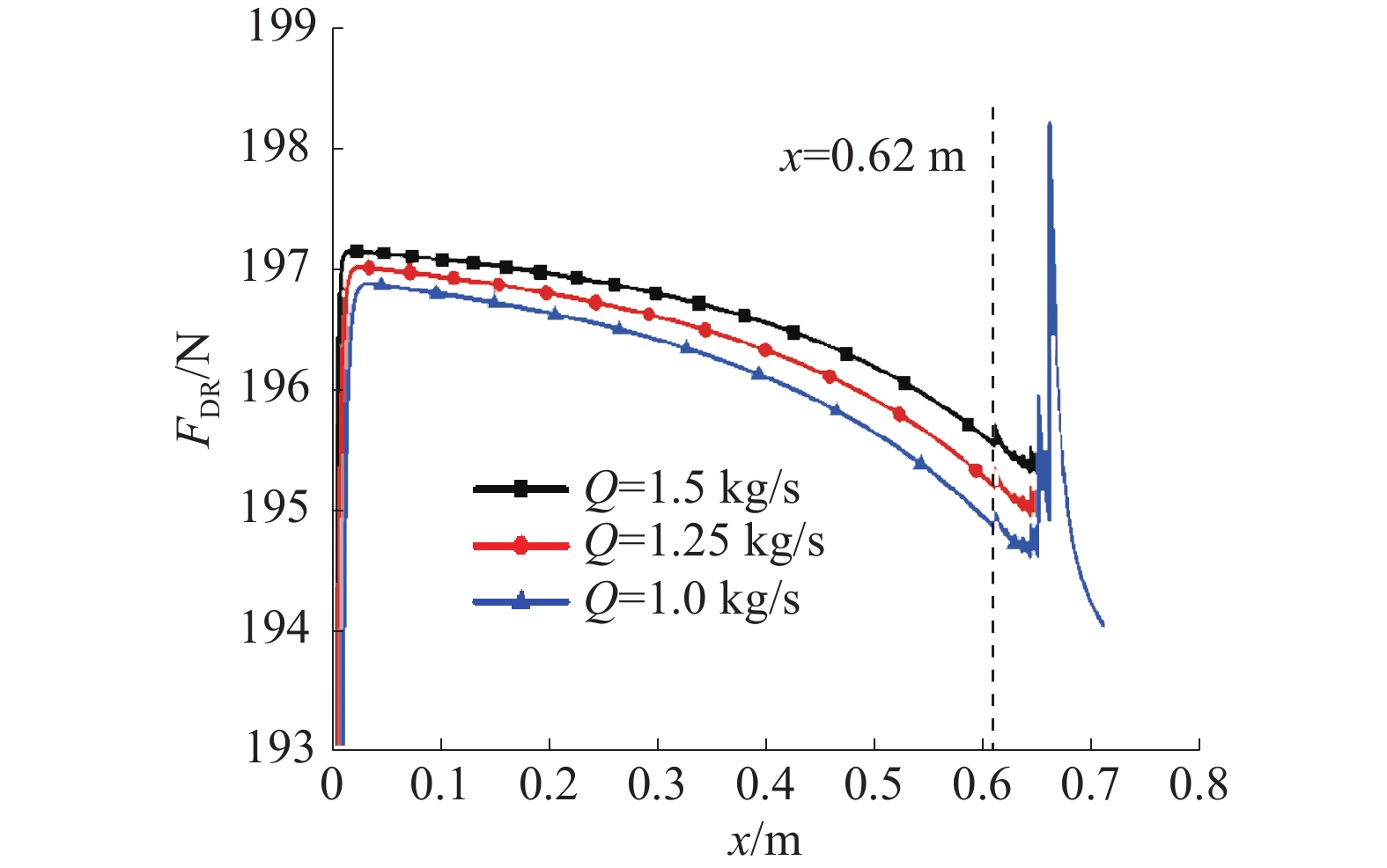Download: 图 8 定流量下移动体受力随位置变化关系

5 PSS结构敏感性分析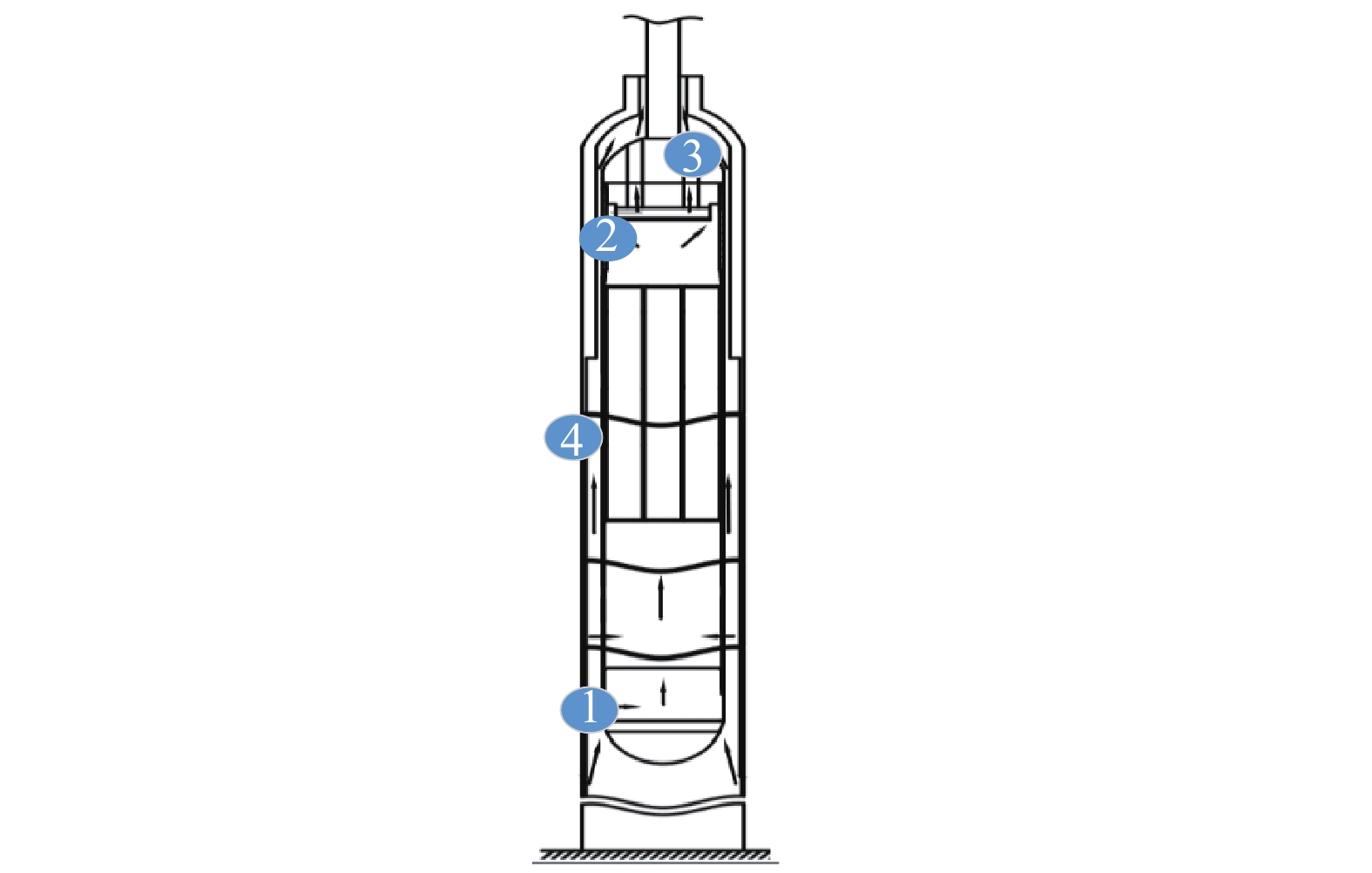Download: 图 9 PSS结构

1)移动体下节流孔的尺寸敏感性计算结果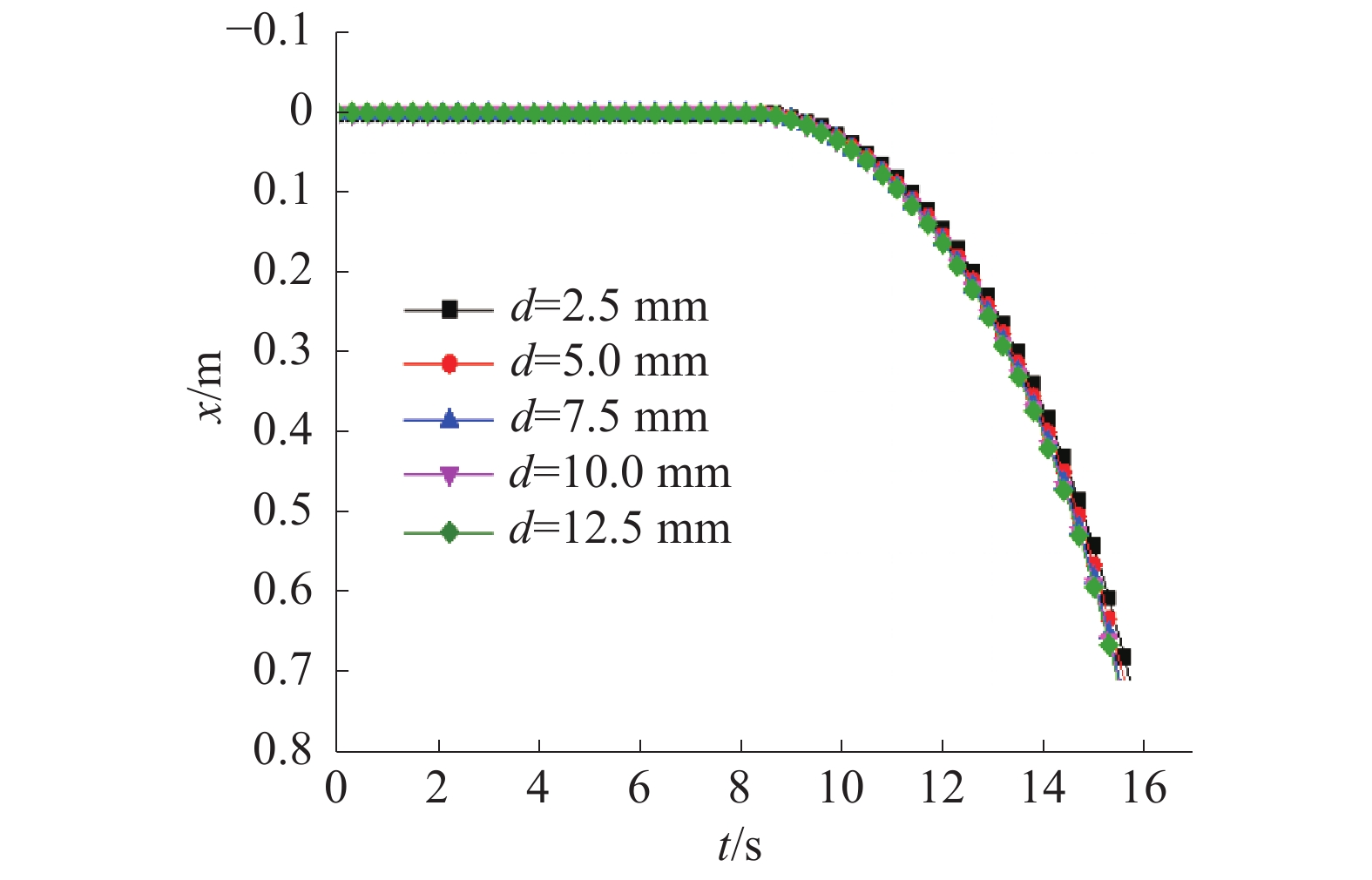Download: 图 10 不同尺寸下位移随时间变化曲线

2)移动体上节流孔的尺寸敏感性计算结果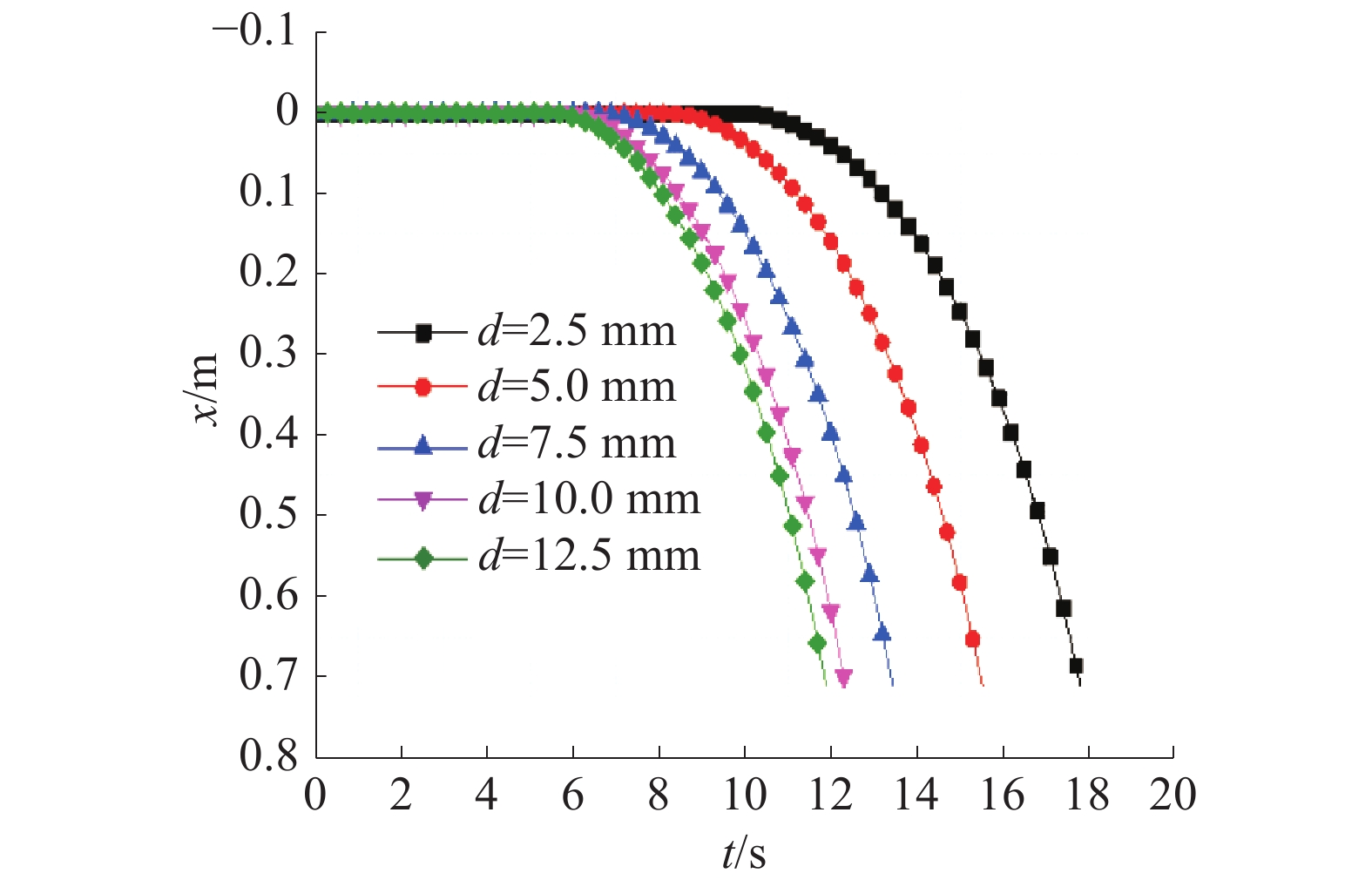Download: 图 11 不同尺寸下位移随时间变化曲线

3)移动体上出口腰型孔尺寸的敏感性计算结果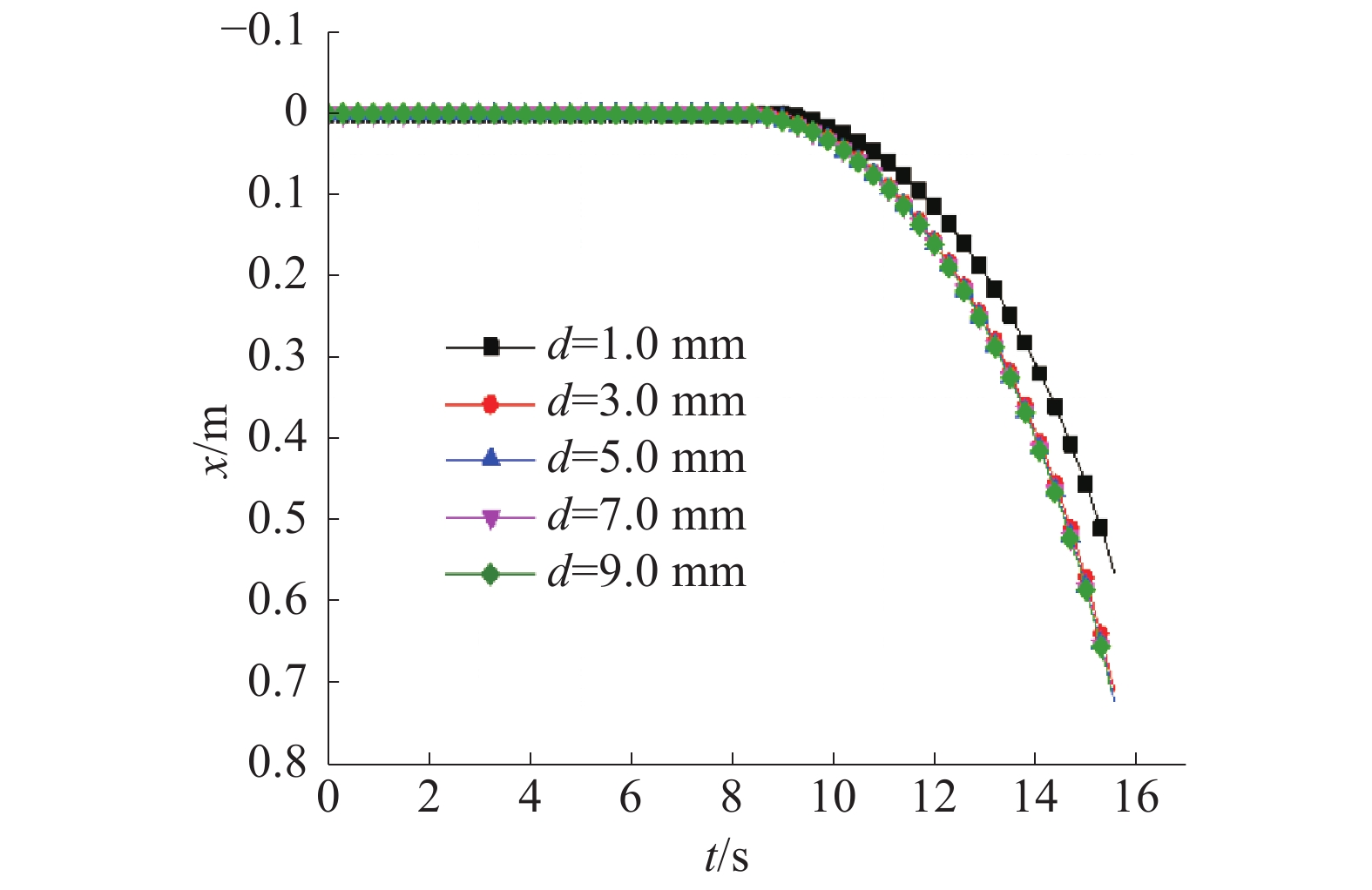Download: 图 12 不同尺寸下位移随时间变化曲线

4)移动体−外套管间环缝宽度敏感性计算结果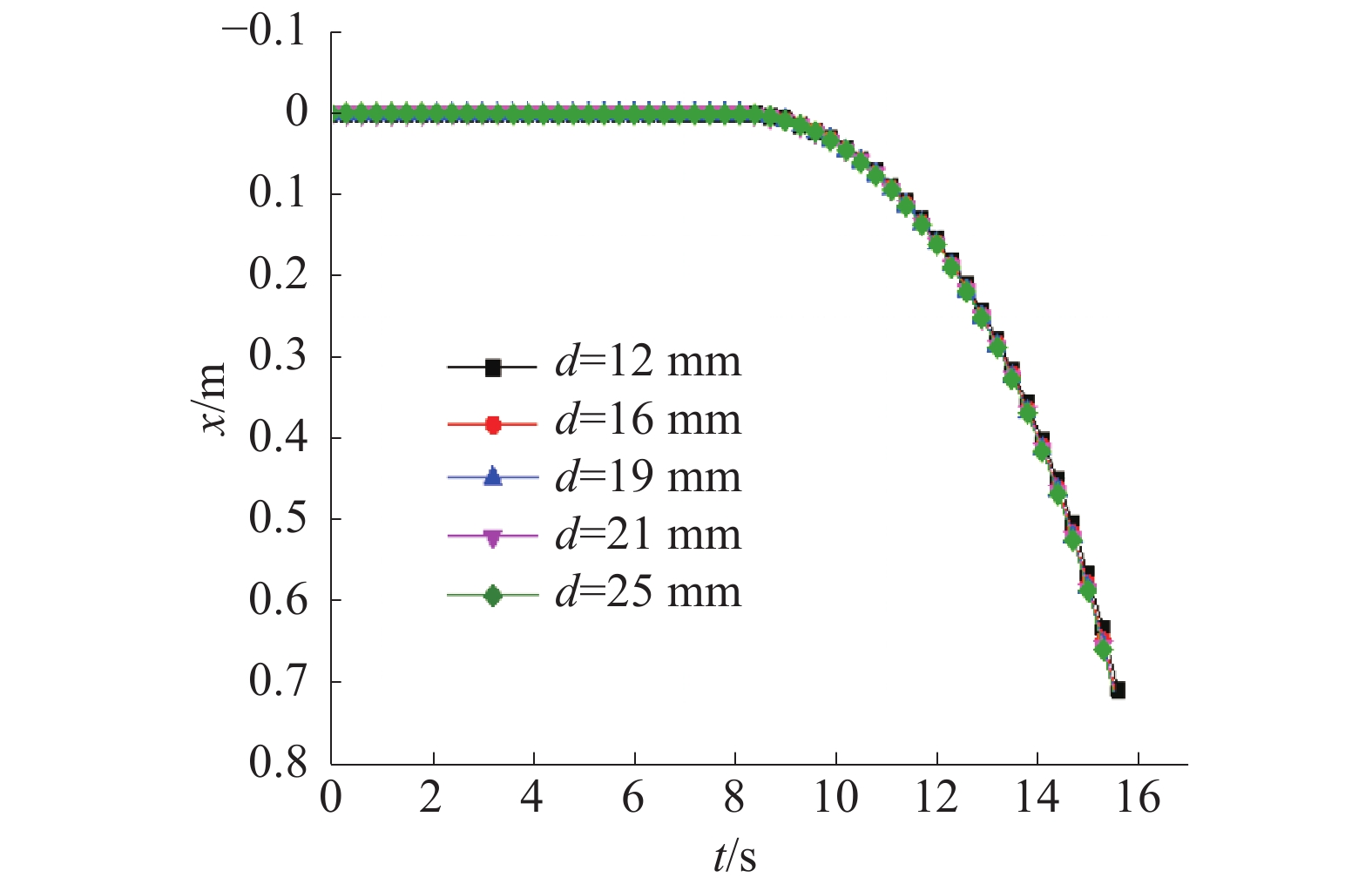Download: 图 13 不同尺寸下位移随时间变化曲线
6 结论

  徐銤, 杨红义. 钠冷快堆及其安全特性[J]. 物理, 2016, 45(9): 561-568. (0)  YOKOO T, OHTA H. ULOF and UTOP analyses of a large metal fuel FBR core using a detailed calculation system[J]. Journal of nuclear science and technology, 2001, 38(6): 444-452. DOI:10.1080/18811248.2001.9715052 (0)  胡文军, 任丽霞, 李政昕, 等. 池式钠冷快堆非能动停堆技术方案研究[J]. 核科学与工程, 2014, 34(1): 23-27. (0)  黄晨. 快堆非能动停堆装置的发展[J]. 中国原子能科学研究院年报, 2006(1): 10-11. (0)  罗志昌, 何绍德. 流体管道系统瞬态流动的网络分析方法[J]. 北京工业大学学报, 1984, 10(1): 1-12. (0)  BABU V R, THANIGAIYARASU G, CHELLAPANDI P. Mathematical modelling of performance of safety rod and its drive mechanism in sodium cooled fast reactor during scram action[J]. Nuclear engineering and design, 2014, 278: 601-617. DOI:10.1016/j.nucengdes.2014.08.015 (0)  BREZINSEK S, WIESEN S, BORODIN D, et al. Quasi-steady-state plasma operation in the Be/W material mix: from the JET tokamak to the ITER reactor[C]//16th International Workshop on Plasma Edge Theory in Fusion Devices (PET16). Marseille, France, 2017. (0)  Bagdasarov Y E, Buksha Y K, Voznesenski R M, et al. Development of passive safety devices for sodium-cooled fast reactors[R]. 1996. (0)  单建强, 王武军, 王学容, 等. 钠冷快堆无保护失流事故和安全特性研究[J]. 核科学与工程, 2002, 22(2): 113-118. DOI:10.3321/j.issn:0258-0918.2002.02.004 (0)  LOEWEN E P, CUNDIFF W M, SAHA P, et al. Passive shutdown system and method of operating a liquid metal cooled reactor using the same[P]. U. S. Patent 9, 911, 513, 2018−03−06. (0)  FUJIMOTO H, SHINOHARA H, AKIZUKI Y, et al. Reactor shutdown system[P]. U. S. Patent 9, 589, 683, 2017−03−07. (0)  KIM T K, JANG J D, PARK J S, et al. Manual valve of hydraulic pressure control system for automatic transmission[P]. U. S. Patent 6, 440, 028, 2002−08−27. (0)